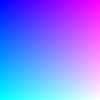# create_image_from_numpy()#

Convert a numpy array into a Py5Image object.

## Examples#```import numpy as np

def setup():
r = np.linspace(0, 255, num=py5.width).reshape(1, -1)
g = np.linspace(0, 255, num=py5.height).reshape(-1, 1)
b = 255
img = py5.create_image_from_numpy(rgb, 'RGB')
py5.image(img, 0, 0)
```

## Description#

Convert a numpy array into a Py5Image object. The numpy array must have 3 dimensions and the array’s `dtype` must be `np.uint8`. The size of `array`’s first and second dimensions will be the image’s height and width, respectively. The third dimension is for the array’s color channels.

The `bands` parameter is used to interpret the `array`’s color channel dimension (the array’s third dimension). It can be one of `'L'` (single-channel grayscale), `'ARGB'`, `'RGB'`, or `'RGBA'`. If there is no alpha channel, `array` is assumed to have no transparency. If the `bands` parameter is `'L'`, `array`’s third dimension is optional.

The caller can optionally pass an existing Py5Image object to put the image data into using the `dst` parameter. This can have performance benefits in code that would otherwise continuously create new Py5Image objects. The array’s width and height must match that of the recycled Py5Image object.

## Signatures#

```create_image_from_numpy(
array: npt.NDArray[np.uint8],  # numpy image array
bands: str = "ARGB",  # color channels in array
*,
dst: Py5Image = None  # existing Py5Image object to put the image data into
) -> Py5Image
```

Updated on September 01, 2022 16:36:02pm UTC